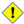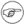On-line GuidesAll GuideseBook StoreiOS / AndroidLinux for BeginnersOffice ProductivityLinux InstallationLinux SecurityLinux UtilitiesLinux VirtualizationLinux KernelSystem/Network AdminProgrammingScripting LanguagesDevelopment ToolsWeb DevelopmentGUI Toolkits/DesktopDatabasesMail SystemsopenSolarisEclipse DocumentationTechotopia.comVirtuatopia.comAnswertopia.comHow To GuidesVirtualizationGeneral System AdminLinux SecurityLinux FilesystemsWeb ServersGraphics & DesktopPC HardwareWindowsProblem SolutionsPrivacy Policy# 8.1. Operators

assignment

variable assignment

Initializing or changing the value of a variable

=

All-purpose assignment operator, which works for both arithmetic and string assignments.

 ```var=27 category=minerals # No spaces allowed after the "=".```Do not confuse the "=" assignment operator with the = test operator.

 ```# = as a test operator if [ "\$string1" = "\$string2" ] # if [ "X\$string1" = "X\$string2" ] is safer, # to prevent an error message should one of the variables be empty. # (The prepended "X" characters cancel out.) then command fi```

arithmetic operators

+

plus

-

minus

*

multiplication

/

division

**

exponentiation
 ```# Bash, version 2.02, introduced the "**" exponentiation operator. let "z=5**3" echo "z = \$z" # z = 125```

%

modulo, or mod (returns the remainder of an integer division operation)

 ```bash\$ expr 5 % 3 2 ```
5/3 = 1 with remainder 2

This operator finds use in, among other things, generating numbers within a specific range (see Example 9-24 and Example 9-27) and formatting program output (see Example 26-15 and Example A-6). It can even be used to generate prime numbers, (see Example A-16). Modulo turns up surprisingly often in various numerical recipes.

Example 8-1. Greatest common divisor

 ```#!/bin/bash # gcd.sh: greatest common divisor # Uses Euclid's algorithm # The "greatest common divisor" (gcd) of two integers #+ is the largest integer that will divide both, leaving no remainder. # Euclid's algorithm uses successive division. # In each pass, #+ dividend <--- divisor #+ divisor <--- remainder #+ until remainder = 0. #+ The gcd = dividend, on the final pass. # # For an excellent discussion of Euclid's algorithm, see # Jim Loy's site, https://www.jimloy.com/number/euclids.htm. # ------------------------------------------------------ # Argument check ARGS=2 E_BADARGS=65 if [ \$# -ne "\$ARGS" ] then echo "Usage: `basename \$0` first-number second-number" exit \$E_BADARGS fi # ------------------------------------------------------ gcd () { dividend=\$1 # Arbitrary assignment. divisor=\$2 #+ It doesn't matter which of the two is larger. # Why not? remainder=1 # If uninitialized variable used in loop, #+ it results in an error message #+ on the first pass through loop. until [ "\$remainder" -eq 0 ] do let "remainder = \$dividend % \$divisor" dividend=\$divisor # Now repeat with 2 smallest numbers. divisor=\$remainder done # Euclid's algorithm } # Last \$dividend is the gcd. gcd \$1 \$2 echo; echo "GCD of \$1 and \$2 = \$dividend"; echo # Exercise : # -------- # Check command-line arguments to make sure they are integers, #+ and exit the script with an appropriate error message if not. exit 0```
+=

"plus-equal" (increment variable by a constant)

let "var += 5" results in var being incremented by 5.

-=

"minus-equal" (decrement variable by a constant)

*=

"times-equal" (multiply variable by a constant)

let "var *= 4" results in var being multiplied by 4.

/=

"slash-equal" (divide variable by a constant)

%=

"mod-equal" (remainder of dividing variable by a constant)

Arithmetic operators often occur in an expr or let expression.

Example 8-2. Using Arithmetic Operations

 ```#!/bin/bash # Counting to 11 in 10 different ways. n=1; echo -n "\$n " let "n = \$n + 1" # let "n = n + 1" also works. echo -n "\$n " : \$((n = \$n + 1)) # ":" necessary because otherwise Bash attempts #+ to interpret "\$((n = \$n + 1))" as a command. echo -n "\$n " (( n = n + 1 )) # A simpler alternative to the method above. # Thanks, David Lombard, for pointing this out. echo -n "\$n " n=\$((\$n + 1)) echo -n "\$n " : \$[ n = \$n + 1 ] # ":" necessary because otherwise Bash attempts #+ to interpret "\$[ n = \$n + 1 ]" as a command. # Works even if "n" was initialized as a string. echo -n "\$n " n=\$[ \$n + 1 ] # Works even if "n" was initialized as a string. #* Avoid this type of construct, since it is obsolete and nonportable. # Thanks, Stephane Chazelas. echo -n "\$n " # Now for C-style increment operators. # Thanks, Frank Wang, for pointing this out. let "n++" # let "++n" also works. echo -n "\$n " (( n++ )) # (( ++n ) also works. echo -n "\$n " : \$(( n++ )) # : \$(( ++n )) also works. echo -n "\$n " : \$[ n++ ] # : \$[ ++n ]] also works echo -n "\$n " echo exit 0```Integer variables in Bash are actually signed long (32-bit) integers, in the range of -2147483648 to 2147483647. An operation that takes a variable outside these limits will give an erroneous result.
 ```a=2147483646 echo "a = \$a" # a = 2147483646 let "a+=1" # Increment "a". echo "a = \$a" # a = 2147483647 let "a+=1" # increment "a" again, past the limit. echo "a = \$a" # a = -2147483648 # ERROR (out of range)```

As of version 2.05b, Bash supports 64-bit integers.Bash does not understand floating point arithmetic. It treats numbers containing a decimal point as strings.
 ```a=1.5 let "b = \$a + 1.3" # Error. # t2.sh: let: b = 1.5 + 1.3: syntax error in expression (error token is ".5 + 1.3") echo "b = \$b" # b=1```
Use bc in scripts that that need floating point calculations or math library functions.

bitwise operators. The bitwise operators seldom make an appearance in shell scripts. Their chief use seems to be manipulating and testing values read from ports or sockets. "Bit flipping" is more relevant to compiled languages, such as C and C++, which run fast enough to permit its use on the fly.

bitwise operators

<<

bitwise left shift (multiplies by 2 for each shift position)

<<=

"left-shift-equal"

let "var <<= 2" results in var left-shifted 2 bits (multiplied by 4)

>>

bitwise right shift (divides by 2 for each shift position)

>>=

"right-shift-equal" (inverse of <<=)

&

bitwise and

&=

"bitwise and-equal"

|

bitwise OR

|=

"bitwise OR-equal"

~

bitwise negate

!

bitwise NOT

^

bitwise XOR

^=

"bitwise XOR-equal"

logical operators

&&

and (logical)

 ```if [ \$condition1 ] && [ \$condition2 ] # Same as: if [ \$condition1 -a \$condition2 ] # Returns true if both condition1 and condition2 hold true... if [[ \$condition1 && \$condition2 ]] # Also works. # Note that && operator not permitted within [ ... ] construct.```&& may also, depending on context, be used in an and list to concatenate commands.
||

or (logical)

 ```if [ \$condition1 ] || [ \$condition2 ] # Same as: if [ \$condition1 -o \$condition2 ] # Returns true if either condition1 or condition2 holds true... if [[ \$condition1 || \$condition2 ]] # Also works. # Note that || operator not permitted within [ ... ] construct.```Bash tests the exit status of each statement linked with a logical operator.

Example 8-3. Compound Condition Tests Using && and ||

 ```#!/bin/bash a=24 b=47 if [ "\$a" -eq 24 ] && [ "\$b" -eq 47 ] then echo "Test #1 succeeds." else echo "Test #1 fails." fi # ERROR: if [ "\$a" -eq 24 && "\$b" -eq 47 ] #+ attempts to execute ' [ "\$a" -eq 24 ' #+ and fails to finding matching ']'. # # Note: if [[ \$a -eq 24 && \$b -eq 24 ]] works. # The double-bracket if-test is more flexible #+ than the single-bracket version. # (The "&&" has a different meaning in line 17 than in line 6.) # Thanks, Stephane Chazelas, for pointing this out. if [ "\$a" -eq 98 ] || [ "\$b" -eq 47 ] then echo "Test #2 succeeds." else echo "Test #2 fails." fi # The -a and -o options provide #+ an alternative compound condition test. # Thanks to Patrick Callahan for pointing this out. if [ "\$a" -eq 24 -a "\$b" -eq 47 ] then echo "Test #3 succeeds." else echo "Test #3 fails." fi if [ "\$a" -eq 98 -o "\$b" -eq 47 ] then echo "Test #4 succeeds." else echo "Test #4 fails." fi a=rhino b=crocodile if [ "\$a" = rhino ] && [ "\$b" = crocodile ] then echo "Test #5 succeeds." else echo "Test #5 fails." fi exit 0```

The && and || operators also find use in an arithmetic context.

 ```bash\$ echo \$(( 1 && 2 )) \$((3 && 0)) \$((4 || 0)) \$((0 || 0)) 1 0 1 0 ```

miscellaneous operators

,

comma operator

The comma operator chains together two or more arithmetic operations. All the operations are evaluated (with possible side effects), but only the last operation is returned.

 ```let "t1 = ((5 + 3, 7 - 1, 15 - 4))" echo "t1 = \$t1" # t1 = 11 let "t2 = ((a = 9, 15 / 3))" # Set "a" and calculate "t2". echo "t2 = \$t2 a = \$a" # t2 = 5 a = 9```

The comma operator finds use mainly in for loops. See Example 10-12.Published under the terms of the GNU General Public License Design by Interspire